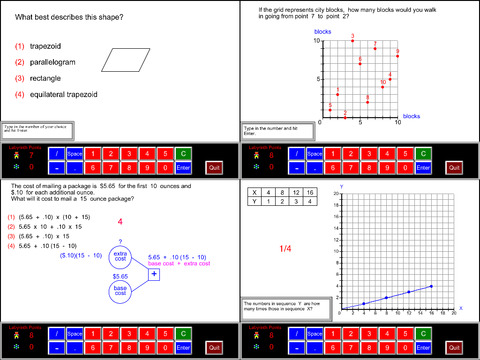## 5th Grade Math Common Core - Lumos Educational App Store41
Price -\$5.99
\$5.99

#### DESCRIPTION:

In fifth grade math the emphasis is on fractions, decimals and volume: (1) developing fluency with addition and subtraction of fractions and multiplication and division of fractions and whole numbers, (2) decimal operations to hundredths and whole number division with 2-digit divisors, and (3) volume.Math Galaxy's Fifth Grade Common Core Math covers all of the Common Core math standards for fifth grade as listed below.The concepts are presented in bite-size pieces with an unlimited supply of practice problems, with numbers chosen randomly.Illustrations are used throughout to reinforce t

#### OVERVIEW:

5th Grade Math Common Core is a free educational mobile app By Math Galaxy.It helps students in grades 5 practice the following standards 5.G.2,5.G.1,5.MD.2.

This page not only allows students and teachers download 5th Grade Math Common Core but also find engaging Sample Questions, Videos, Pins, Worksheets, Books related to the following topics.

1. 5.G.2 : Represent real world and mathematical problems by graphing points in the first quadrant of the coordinate plane, and interpret coordinate values of points in the context of the situation. .

2. 5.G.1 : Use a pair of perpendicular number lines, called axes, to define a coordinate system, with the intersection of the lines (the origin) arranged to coincide with the 0 on each line and a given point in the plane located by using an ordered pair of numbers, called its coordinates. Understand that the first number indicates how far to travel from the origin in the direction of one axis, and the second number indicates how far to travel in the direction of the second axis, with the convention that the names of the two axes and the coordinates correspond (e.g., x-axis and x-coordinate, y-axis and y-coordinate)..

3. 5.MD.2 : Make a line plot to display a data set of measurements in fractions of a unit (1/2, 1/4, 1/8). Use operations on fractions for this grade to solve problems involving information presented in line plots. For example, given different measurements of liquid in identical beakers, find the amount of liquid each beaker would contain if the total amount in all the beakers were redistributed equally..

5

#### STANDARDS:

5.G.2
5.G.1
5.MD.2
5.MD.1
5.NF.2
5.NF.1
5.NBT.1
5.NBT.2
5.OA.2
5.OA.1
5.OA.A.1
5.OA.A.2
5.NF.A.1
5.NF.A.2
5.NBT.A.1
5.NBT.A.2
5.MD.A.1
5.MD.B.2
5.G.A.1
5.G.A.2

Developer: Math Galaxy

Software Version: 1.1

Category: Education

Release Date: July 30,2015### RELATED APPSEdSearch WebSearch